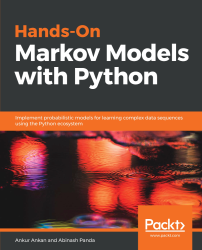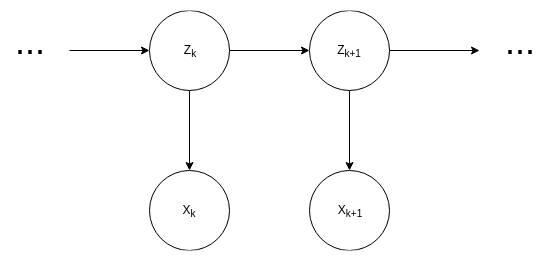•#### Hands-On Markov Models with Python#### Overview of this book

Hidden Markov Model (HMM) is a statistical model based on the Markov chain concept. Hands-On Markov Models with Python helps you get to grips with HMMs and different inference algorithms by working on real-world problems. The hands-on examples explored in the book help you simplify the process flow in machine learning by using Markov model concepts, thereby making it accessible to everyone. Once you’ve covered the basic concepts of Markov chains, you’ll get insights into Markov processes, models, and types with the help of practical examples. After grasping these fundamentals, you’ll move on to learning about the different algorithms used in inferences and applying them in state and parameter inference. In addition to this, you’ll explore the Bayesian approach of inference and learn how to apply it in HMMs. In further chapters, you’ll discover how to use HMMs in time series analysis and natural language processing (NLP) using Python. You’ll also learn to apply HMM to image processing using 2D-HMM to segment images. Finally, you’ll understand how to apply HMM for reinforcement learning (RL) with the help of Q-Learning, and use this technique for single-stock and multi-stock algorithmic trading. By the end of this book, you will have grasped how to build your own Markov and hidden Markov models on complex datasets in order to apply them to projects.
PrefaceFree Chapter
Introduction to the Markov ProcessHidden Markov ModelsState Inference - Predicting the StatesParameter Learning Using Maximum LikelihoodParameter Inference Using the Bayesian ApproachTime Series PredictingNatural Language Processing2D HMM for Image ProcessingMarkov Decision ProcessOther Books You May Enjoy# Backward algorithm

Let's formally define the problem statement for the backward algorithm. In this case, we are trying to compute the probability of observation variables given the current state:

Backward algorithm: P(Xk+1:n|Zk)Figure 3.4: HMM showing two time slices, k and k+1

Similar to what we did in the case of the forward algorithm, we will again try to convert this probability term into a recursive equation in terms of known distributions, so that we can recursively compute the probabilities at different time instances. First, we introduce a new term, Zk+1 in P(Xk+1:n|Zk), using the marginalization rule:Here, we are marginalizing over the Zk+1 variable by summing over all of its possible states, which we have assumed to be m. Now, we can use the product rule of probability to split the preceding equation as:Now, from the d-separation property of our model, we...